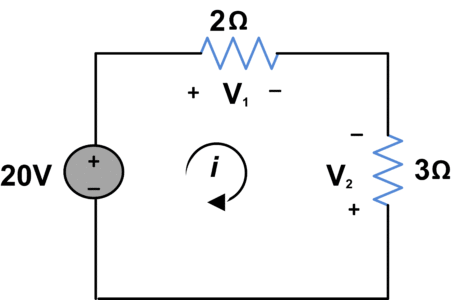Home / Basic Electrical / Kirchhoff’s Voltage Law (KVL)

# Kirchhoff’s Voltage Law (KVL)

Want create site? Find Free WordPress Themes and plugins.

In order to present Kirchhoff’s voltage law, we must introduce the concept of a “loop”.

LoopStarting at any node n in an electric circuit, we form a loop by traversing through elements and returning to the starting node n, and never encountering any other node more than once.

Since energy must be conserved when a charge goes around a loop, the energy given up by the charge equals the energy it gains. The same energy-conservation principle would apply if you carried a rock over a hill and back to where you started.

Kirchhoff’s Voltage Law expresses conservation of energy in terms of voltage rises and drops around a loop in a circuit.

KVL DefinitionThe sum of the voltage drops around any loop equals the sum of the voltage rises.

An alternate statement of KVL can be obtained by considering voltages across elements that are traversed from plus to minus to be Positive in sense and voltages across elements that are traversed from minus to plus to be negative in sense (or vice versa). Under this circumstances, KVL has the following alternative form:

KVL Alternate FormThe algebraic sum of all voltage drops around any loop equals zero.

Specifically, this statement becomes,

$\sum V=0$

By applying this form of KVL to the circuit shown in figure 1, and selecting a traversal from plus to minus to be positive in sense, around the loop, we get

$E-V=0$Figure.1: Kirchhoff’s Voltage Law Circuit

## Kirchhoff’s Voltage Law Solved Example

Find  V1  and  V2 using Kirchhoff’s voltage law.SolutionBy using Ohm’s Law,

${{V}_{1}}=2i$

and
${{V}_{2}}=-3i$

Using KVL;

$-20+{{V}_{1}}-{{V}_{2}}=0$

$-20+2i+3i=0$

$i=4A$

So,

${{V}_{1}}=8V$

${{V}_{2}}=-12V$

## Application of KVL

A very simple application of KVL is to combine all voltage sources in series.In above circuit, we observe that polarity of  V1 and  V2 is same as supply voltage  Vab so we consider them positive voltage while V3 polarity is opposite to supply voltage. So, the final resultant voltage will be;

${{V}_{ab}}={{V}_{1}}+{{V}_{2}}-{{V}_{3}}$

Did you find apk for android? You can find new Free Android Games and apps.Mr. Ahmed Faizan Sheikh, M.Sc. (USA), Research Fellow (USA), a member of IEEE & CIGRE, is a Fulbright Alumnus and earned his Master’s Degree in Electrical and Power Engineering from Kansas State University, USA.

1.